# Planar Triangulations

This post is the first part of a multiple part series covering triangulation and interpolation using Igor Pro 7.

Consider a set of 30 points {xi,yi} sampled from a uniform distribution in the range [-5,5].  You can create such a set using the command:

`Make/o/N=30 xwave=enoise(5), ywave=enoise(5)`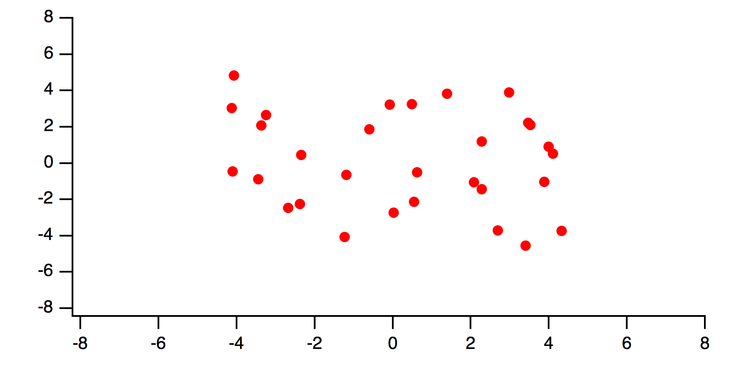Figure 1: a scatter plot of ywave vs xwave.

The convex hull of this set of points is the minimal bounding region so that a line segment connecting any two set points is completely inside the region.  A good way to visualize the convex hull is to think of placing a tight rubber band around the set of points.

You can compute the convex hull for this data set and append it to the graph using the commands:

Convexhull/c xwave,ywave
Setdrawenv xcoord=bottom,ycoord=left
Drawpoly/w=graph0 /ABS 0,0,1,1,W_XHull,W_YHull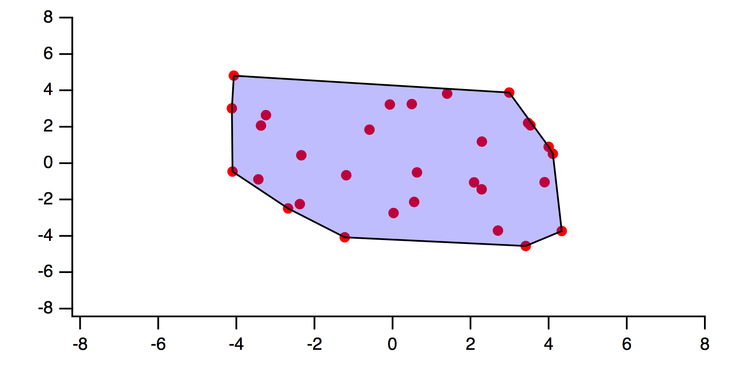Figure 2: the convex hull for the scatter set.  A line segment connecting any two points in the set lies completely inside the shaded region.

Suppose each one of the {xi,yi} pairs is associated with some z-value, zi=f(xi,yi), we might want to know the value of z for an arbitrary {x,y}.  In the absence of additional information we can only make meaningful statements about points inside the convex domain.  To understand the logic of our next steps it helps to first consider a brief 1D analogy:

The following graph shows a set of points y=g(x) sampled at random positions {xi}.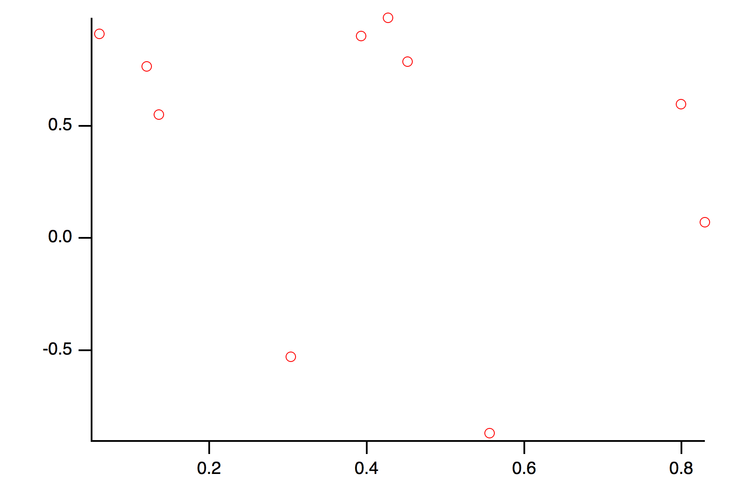Figure 3: a random set of points in the XY plane.

Since we have no further information about g(x) we start by assuming that we can connect nearest points by a straight line (linear approximation).  In practice we first sort the points in ascending x-values and then connect the sequence with straight line segments.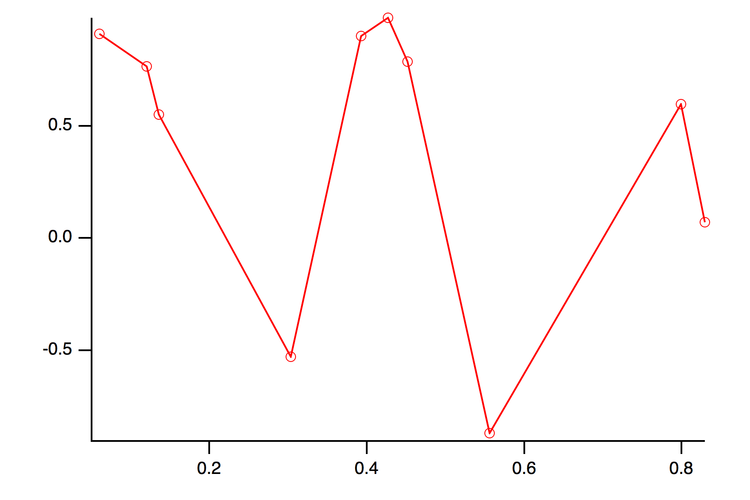Figure 4: The linear approximation obtained by connecting the nearest points by straight lines.

In this case we happen to know that the data were sampled from the blue curve in the graph below.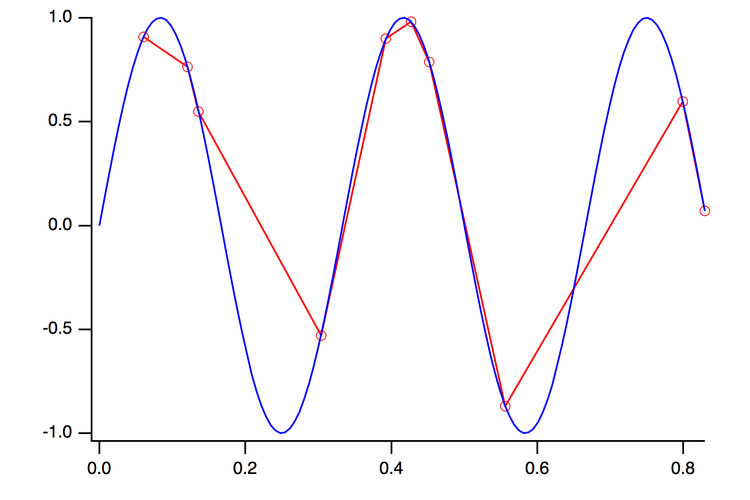Figure 5: The linear approximation and the original data.

Extending the "linear approximation" approach to the 2D scatter example we considered earlier, we need to find a way to identify nearest points in the plane.  Here each group of three nearest (non-collinear) points defines a triangle.  The 2D/3D analogy of sorting and connecting nearest points is known as triangulation.  Although there may be more than one way to subdivide the domain into triangles we focus on the Delaunay triangulation which maxmizes the minimum (triangle) angle and is unique except when the 4 nearest points lie on the same circle.  This can happen when the samples are vertices of a rectangle.  I will discuss this case in the next blog-post.

For the purpose of this example we will assume that the random set xwave and ywave represent sampling from a gaussian function:

Make/o/N=30 zwave               // create the third wave
zwave=gauss(xwave[p],0,3,ywave[p],0,3)  // fill it with Gaussian samples

Since some of the following operations require a triplet wave input, we create a triplet wave from the data using the command:

`Concatenate {xwave,ywave,zwave},tripletWave`

You can now compute and display the triangulation of the data using:

AppendXYZContour tripletWave
ModifyContour tripletWave xymarkers=1,boundary=1,triangulation=1;DelayUpdate
ModifyContour tripletWave autoLevels={*,*,0}

You can also obtain the triangulation data  in a form suitable for display in Gizmo using the ImageInterpolate operation with the /CMSH flag.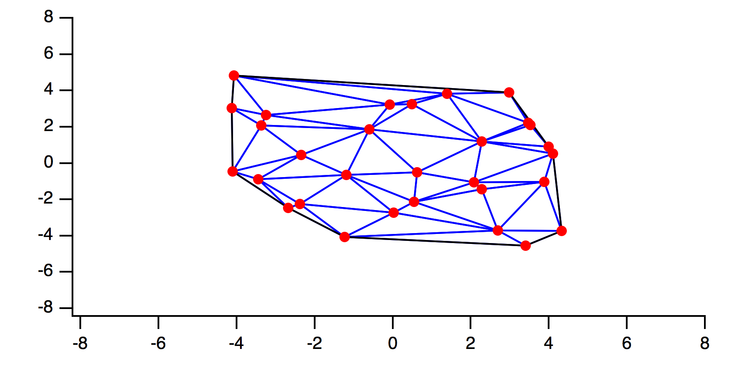Figure 6: Delaunay triangulation of the scatter data set in the XY plane.  The triangulation depends only on the the X and Y coordinates.

Using this triangulation you could obtain an approximate z-value for any point inside the convex domain by locating the triangle that the point falls into and linearly interpolating between the 3 z-values at the vertices.

The triangular (linearly interpolated) surface looks like this (Gizmo top view):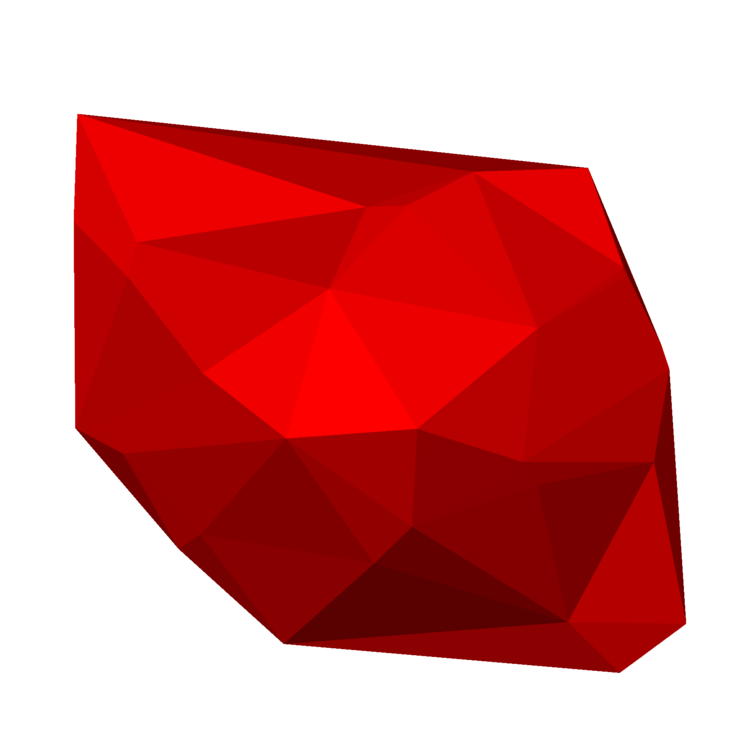Figure 7:  Gizmo plot of the filled triangle surface.  This is the 2D analogy of the linear approximation.

A smoother representation of the surface can be obtained using Voronoi interpolation:

`ImageInterpolate/CMSH/RESL={300,300} voronoi tripletWave `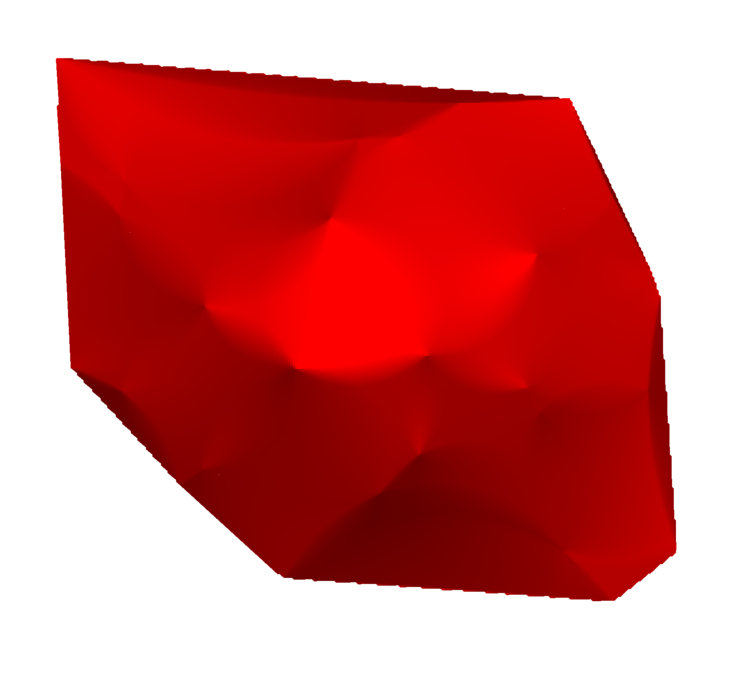Figure 8: Voronoi interpolated surface.

Although this surface is smoother, the z-values at all the original data points are actually unchanged, as shown below: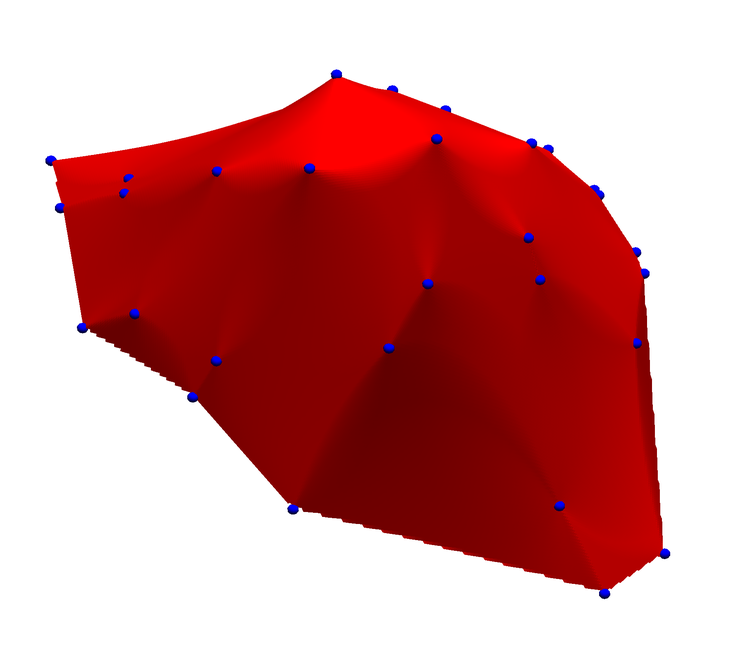Figure 9: The Voronoi interpolated surface with blue markers indicating the positions of the original data.  The interpolated surface clearly passes through all the original data points.

The same data can be displayed as an image plot.  The figure below shows the interpolated image together with the underlying triangulation.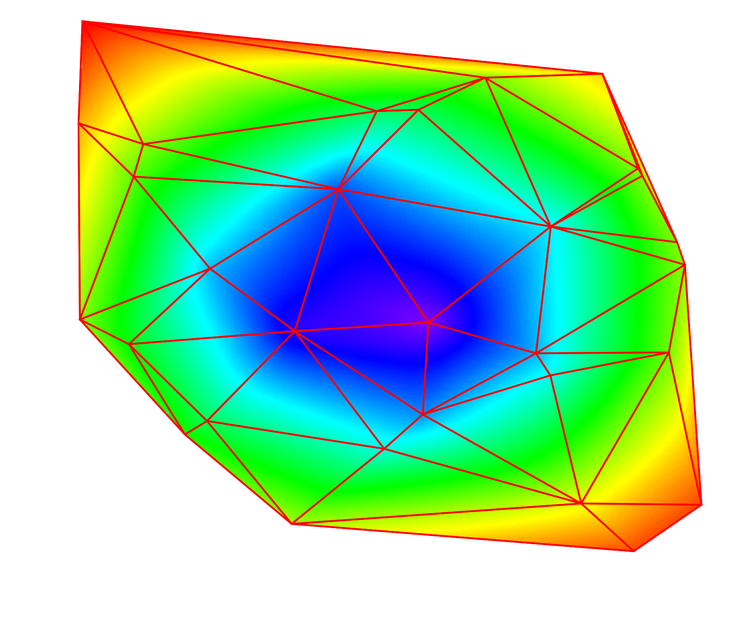Figure 10:  An image plot of the Voronoi interpolated data with the triangulation overlay.

Note that the Voronoi interpolation shown above is different from the linear interpolation.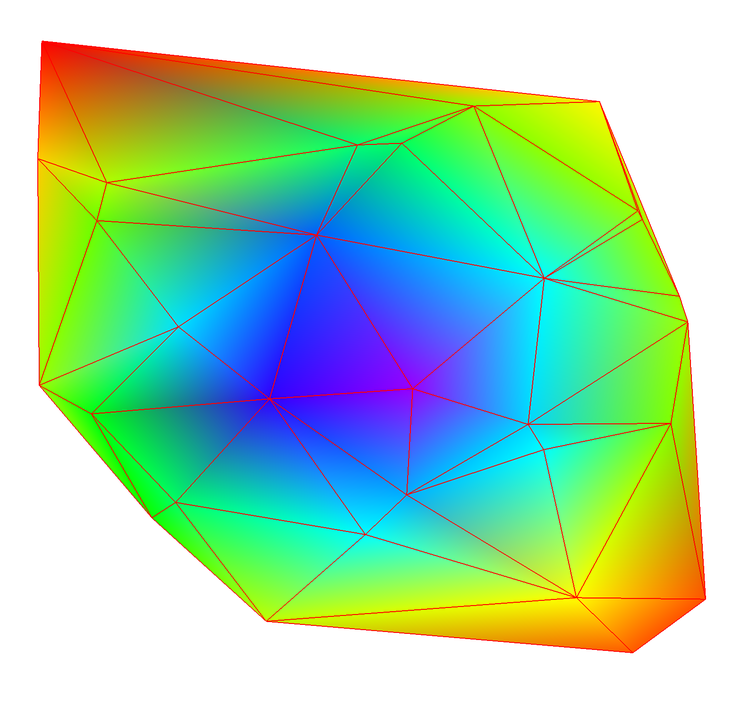Figure 11: linear interpolation of the triangulation data.  The graph was created in Gizmo using OpenGL color interpolation between the three vertices of each triangle.

You can also examine the effect of the Voronoi interpolation by comparing contours of constant value.  We start with drawing a contour by connecting the intersections of the constant level with the edges of the triangulation (left image).  We then compute the same contour for the result of the Voronoi interpolation (right image).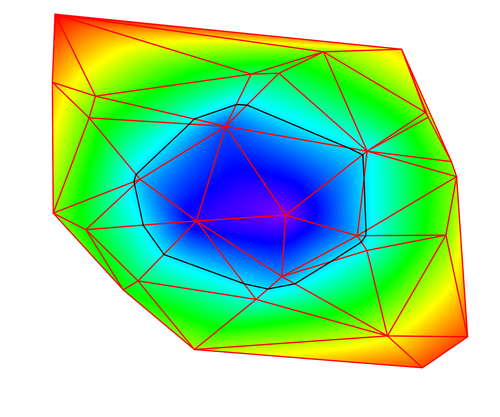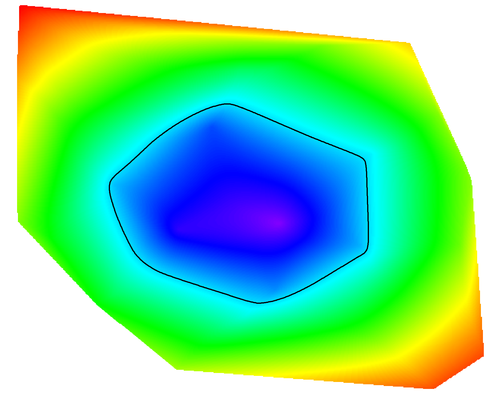As you can see, the black contour on the left consists of straight line segments between triangle edges while the black contour line on the right is fairly smooth (rounded).  On the other hand, the boundary of the convex hull is not as smooth on the right.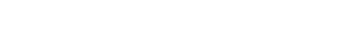ForumSupportGallery